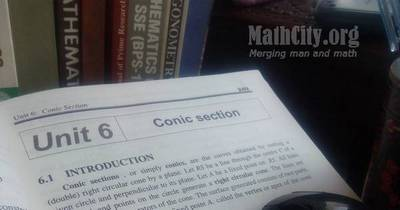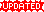# Unit 06: Conic SectionNotes (Solutions) of Unit 06: Conic Section, Calculus and Analytic Geometry, MATHEMATICS 12 (Mathematics FSc Part 2 or HSSC-II), Punjab Text Book Board Lahore. You can view online or download PDF. To view PDF, you must have PDF Reader installed on your system and it can be downloaded from Software section.

• Introduction
• Equation of a Circle
• General form of an Equation of a Circle
• Equation of a Circle Determind by given Conditions
• Exercise 6.1
• Tangents and Normals
• Length of the Tangent to a Circle (Tangential distance)
• Exercise 6.1
• Analytic Proofs of Important Properties of a Circle
• Exercise 6.3
• Parabola
• General form of an Equation of a Parabola
• Other Standard Parabolas
• Graph the Parabola
• Exercise 6.4
• Ellipse and its Elements
• Standard form of an Ellipse
• Graph of an Ellipse
• Exercise 6.5
• Hyperbola and its Elements
• Standard Equation of Hyperbola
• Graph of the Hyperbola
• Exercise 6.6
• Tangents and Normals
• Intersection of two Conics
• Exercise 6.7
• Translation and Rotation of Axes
• Exercise 6.8
• The General Equation of Second Degree
• Classification of Conics by the Discriminant
• Exercise 6.9
• Exercise 6.2 | View Online | Download PDF (189kB)### Here are previous and next chapters

• fsc/fsc_part_2_solutions/ch06